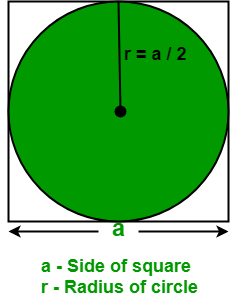Related Articles

# Program to calculate area of an Circle inscribed in a Square

• Last Updated : 27 Apr, 2021

Given the side of a square. The task is to find the area of an inscribed circle in a square.
Examples:

```Input : a = 8
Output : Area of an inscribed circle: 50.24

Input : a = 12.04
Output : Area of an inscribed circle: 113.795```

Given a square i.e. all sides of a square are of equal length and all four angles are 90 degrees. Below diagram depicts an inscribed circle in a square.Properties of an inscribed circle in a square:

• The diameter of an inscribed circle in a square is equal to the length of the side of a square.
• With at least one measure of the circle or the square, the area and the perimeter of the square can be calculated in which the circle is inscribed.
• The center of the square and the center of the circle lie at a same point.
• when at least one measure of the circle or the square is given, the circumference and area of the circle can be calculated.

Formula to find the area of an inscribed circle:∏ /{4} a2

where a is the side of a square in which a circle is inscribed.

How does the formula works?

Assume a is the side of a square and we know that a square has 4 sides.

Area of a circle = ∏ r2

where r is the radius of a circle and area of a square = a2

Therefore, the area of an inscribed circle in a square =  ∏ r2

Now, put r = a / 2

So, the area of an inscribed circle in a square ∏ /{4} a2

## C++

 `// C++ Program to find the area of``// an inscribed circle in a square.``#include``#define PI 3.14``using` `namespace` `std;` `// Function to find area of an``// inscribed circle in a square.``float` `areaOfInscribedCircle(``float` `a)``{``    ``return` `( PI / 4 ) * a * a;``}` `// Driver's code``int` `main()``{``    ``float` `a = 8;``    ` `    ``cout << ``"Area of an inscribed circle: "``        ``<< areaOfInscribedCircle(a);``        ` `    ``return` `0;``}`

## Java

 `// Java Program to find the area of``// an inscribed circle in a square.``import` `java.io.*;` `class` `GFG {``    ` `    ``static` `double` `PI = ``3.14``;``    ` `    ``// Function to find area of an``    ``// inscribed circle in a square.``    ``static` `double` `areaOfInscribedCircle(``float` `a)``    ``{``        ``return` `( PI / ``4` `) * a * a;``    ``}` `    ``// Driver code``    ``public` `static` `void` `main (String[] args)``    ``{``        ``float` `a = ``8``;``    ` `        ``System.out.println(``"Area of an inscribed"``        ``+ ``" circle: "` `+ areaOfInscribedCircle(a));``    ``}``}`

## Python3

 `# Python Program to find the area of``# an inscribed circle in a square.``    ` `PI ``=` `3.14``    ` `# Function to find area of an``# inscribed circle in a square.``def` `areaOfInscribedCircle(a):``    ``return` `( PI ``/` `4` `) ``*` `a ``*` `a` `# Driver code``a ``=` `8``print``(``"Area of an inscribed circle:"``,``round``(areaOfInscribedCircle(a), ``2``))`

## C#

 `// C# Program to find the``// area of an inscribed``// circle in a square.``using` `System;` `class` `GFG``{``    ``static` `double` `PI = 3.14;``    ` `    ``// Function to find area``    ``// of an inscribed circle``    ``// in a square.``    ``static` `double` `areaOfInscribedCircle(``float` `a)``    ``{``        ``return` `(PI / 4 ) * a * a;``    ``}` `    ``// Driver code``    ``public` `static` `void` `Main ()``    ``{``        ``float` `a = 8;``    ` `        ``Console.WriteLine(``"Area of an inscribed"` `+``                                    ``" circle: "` `+``                        ``areaOfInscribedCircle(a));``    ``}``}` `// This code is contributed``// by anuj_6`

## PHP

 ``

## Javascript

 ``
Output
`Area of an inscribed circle: 50.24`

Attention reader! Don’t stop learning now. Get hold of all the important mathematical concepts for competitive programming with the Essential Maths for CP Course at a student-friendly price. To complete your preparation from learning a language to DS Algo and many more,  please refer Complete Interview Preparation Course.

My Personal Notes arrow_drop_up